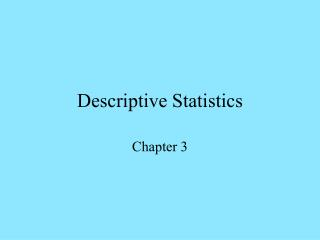DownloadDownload PresentationDescriptive Statistics

# Descriptive Statistics

Télécharger la présentation## Descriptive Statistics

- - - - - - - - - - - - - - - - - - - - - - - - - - - E N D - - - - - - - - - - - - - - - - - - - - - - - - - - -
##### Presentation Transcript

1. Descriptive Statistics Chapter 3

2. Numerical Scales Nominal scale-Uses numbers for identification (student ID numbers) Ordinal scale- Uses numbers for ranking (order of winners in a race) Interval scale- Uses numbers for ranking when units are equidistant (measuring temperature, but no absolute zero)) Ratio scale-This scale has qualities of equidistant units and absolute zero (age).

3. Descriptive Statistics Descriptive Statistics- Statistics used to organize and describe data Measures of Central Tendency- Statistic methods for observing how data cluster around the mean Normal Distribution-A symmetrical distribution with a single numerical representation for mean, median, and mode Measures of Dispersion-Statistical methods for observing how data spread from the mean.

4. Counting the Data-Frequency Look at the set of data that follows on the next slide. Each time a score occurred, a tally mark was made to count Which number most likely represents the average score? Which number is the most frequently occurring score?

5. Frequency Distribution Scores 100 99 98 94 90 89 88 82 75 74 68 60 Tally 1 1 11 11 1111 1111 11 1111 1111 1111 1 11 1 1 1 Frequency 1 1 2 2 5 7 10 6 2 1 1 1 Average Score? Most Frequent Score? 88 88

6. This frequency count represents data that closely represent a normal distribution. Tally 1 1 11 11 1111 1111 11 1111 1111 1111 1 11 1 1 1

7. Frequency Polygons Data 100 89 99 89 98 89 98 89 94 88 94 88 90 75 90 75 90 74 90 68 90 60 5 4 3 2 1 60 68 74 75 88 89 90 94 98 99 100 Scores

8. Mean, Median, and Mode Mean- The arithmetic average of a set of scores Median-The middlemost point in a set of data Mode- The most frequently occurring score in a set of data

9. Mean- To find the mean, simply add the scores and divide by the number of scores in the set of data. 98 + 94 + 88 + 75 = 355 Divide by the number of scores: 355/4 = 88.75

10. Median-The Middlemost point in a set of data Data Set 1 100 99 99 98 97 96 90 88 85 80 79 Data Set 2 100 99 98 97 86 82 78 72 70 68 The median is 84 for this set. 84 represents the middle most point in this set of data. Median 96

11. Mode-The most frequently occurring score in a set of data. Find the modes for the following sets of data: 88 and 87 are both modes for this set of data.This is called a bimodal distribution. Three or more modes represent a multi- modal distribution. Data Set 3 99 89 89 89 89 75 Data set 4 99 88 88 87 87 72 70 Mode: 89

12. Measures of Dispersion Range- Distance between the highest and lowest scores in a set of data. 35 100 - 65 = 35 is the range in this set of scores.

13. Variance-Describes the total amount that a set of scores varies from the mean. 1. Subtract the mean from each score. When the mean for a set of data is 87, subtract 87 from each score.

14. 2. Next-Square each difference- multiply each difference by itself. 13 x 13 = 169 11 x 11 = 121 x 8 = 64 x 4 = 16 -2 x -2 = 4 -7 x -7 = 49 -27x -27 = + 729 100 - 87 = 13 98- 87 = 11 95- 87 = 8 91- 87 = 4 85- 87 = -2 80- 87 = -7 60- 87 = -27 3. Sum these differences 1,152 Sum of squares

15. 4. Divide the sum of squares by the number of scores. 1,152 divided by 7 =164.5714-This number represents the variance for this set of data.

16. Standard Deviation-Represents the typical amount that a score is expected to vary from the mean in a set of data. 5. To find the standard deviation, find the square root of the variance. For this set of data, find the square root of 164.5714. The standard deviation for this set of data Is 12.82 or 13.

17. Percentile Ranks -Scores that express the percentage of students who scored as well as or lower than a given student’s score. A student received a percentile rank of 75 on a recent statewide exam. What does the 75 mean? This indicates that 75% of the students in the same grade or age group made the same score or a lower score. It also means that 25% of this group made the same score or higher than this student.

18. Properties of a Normal Distribution or The “Bell Curve” The mean, median, and mode are represented by the same numerical value. Both sides of the curve are symmetrical Anything that occurs naturally and is measurable will be distributed in a normal distribution when sufficient data are collected.

19. The Normal Curve

20. Bell Curve

21. The Normal Distribution and Norm-Referenced Testing Norm-referenced tests compare students with their age or grade peers. Scores on these tests are compared with the national sample to determine if a specific student scored above or below the level expected for their age or grade. When a score is significantly above or below the level expected for the age or grade, it may indicate that the student requires educational interventions.